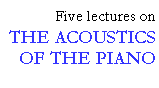## Simulation of changes in the design

```
```

I will end my presentation with a short discussion of how modal analysis can be used to predict the decay times. The modal analysis program models the properties of the real soundboard by a set of hypothesized mathematical resonating systems (with one degree of freedom). Any changes in the properties of the soundboard can be simulated in the computer by changing the values of the mass, the stiffness, and the damping in the measuring points.

The technique is illustrated in Fig. 17, which shows the deflection of a soundboard at its second mode of 90 Hz (upper panel). The largest vibration amplitude is found in the front left section and the smallest around the nodal line (broken line). The nodal line crosses the bridge near a point (0) where one of the bass strings delivers its vibration energy to the soundboard. The small vibration amplitude at that point indicates a high input impedance to the string and consequently a very long decay time of the prompt sound.

```
```Fig. 17. Second mode at 90 Hz of a grand piano soundboard (top), and as modified in the computer model, at 101 Hz (bottom). The change in the modal pattern and frequency is due to a simulated increase in stiffness at the front left corner (shaded area). Nodal lines are marked with broken lines. The circles indicate a point on the bridge where a certain bass string crosses, see text.

```
```

If the stiffness of the front, left corner of the soundboard is increased in the computer model (lower panel, shaded area), the shape of the second mode will change. The frequency will be shifted up to 101 Hz, and the nodal line turned toward the longitudinal direction of the soundboard. The motion at the front left section will decrease as would be expected. In contrast, the vibration amplitude at the point where the bass string crosses the bridge will increase. This means that the early decay time will drop as intended - how much is, however, harder to predict.

This example illustrates how modal analysis followed by computer modifications can assist in the development of pianos, by predicting the general effects of proposed changes in the design. By means of this technique, the need for expensive test prototypes can be minimized, although as yet, not completely excluded.

This lecture is one of Five lectures on the Acoustics of the piano